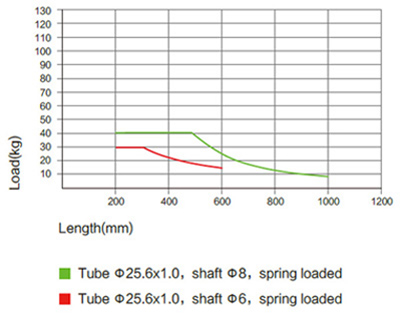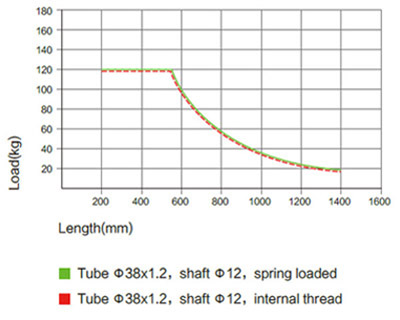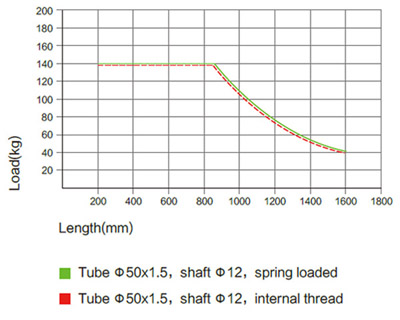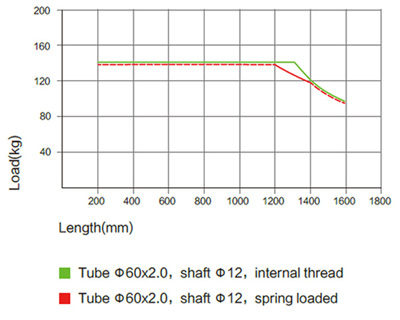Note: Above data shows the static load capacity of the roller for a uniformly distributed load.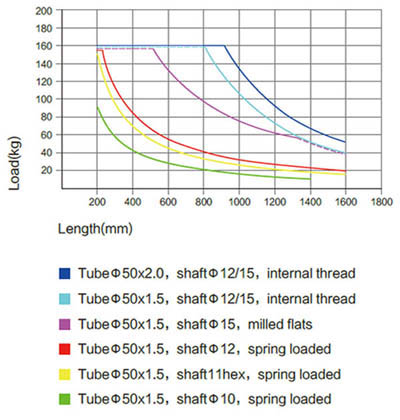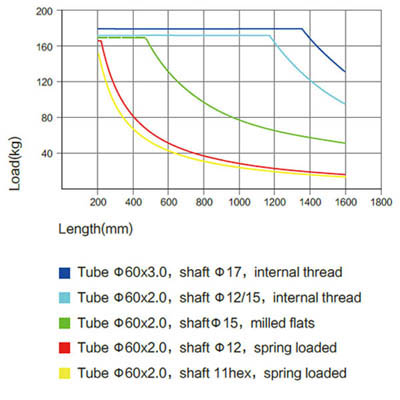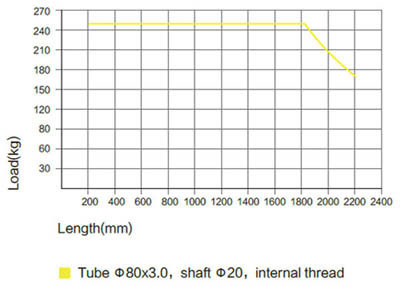Note: Above data shows the static load capacity of the roller for a uniformly distributed load.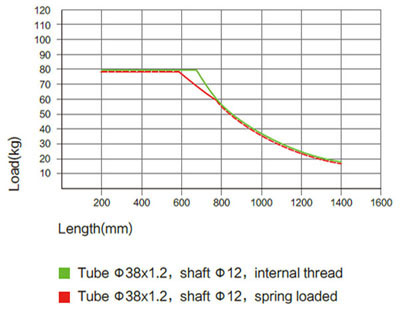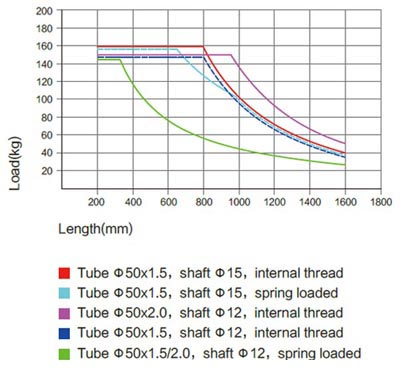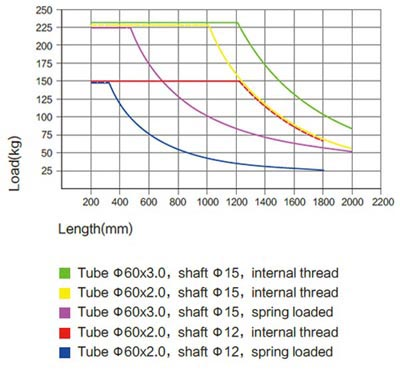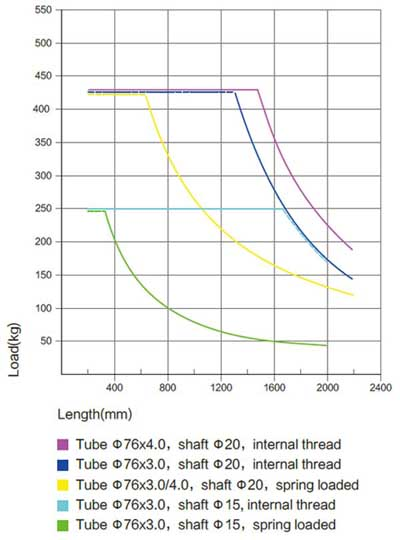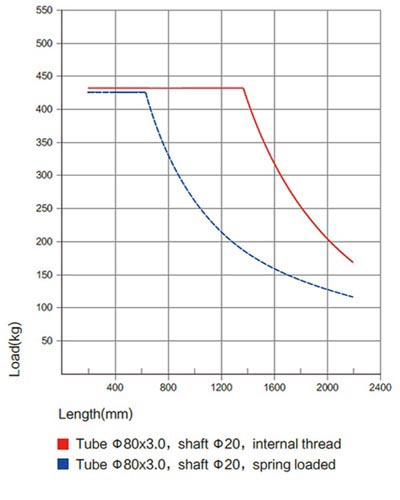Note: Above data shows the static load capacity of the roller for a uniformly distributed load.

2311 / 2321 SERIES LOAD CAPACITY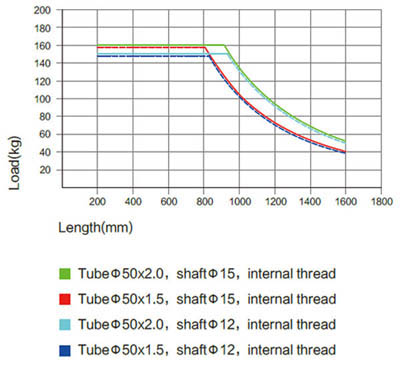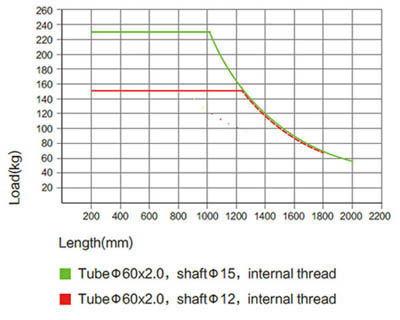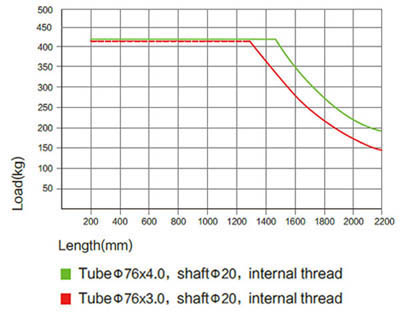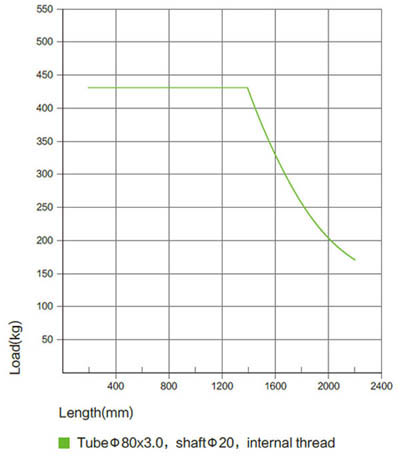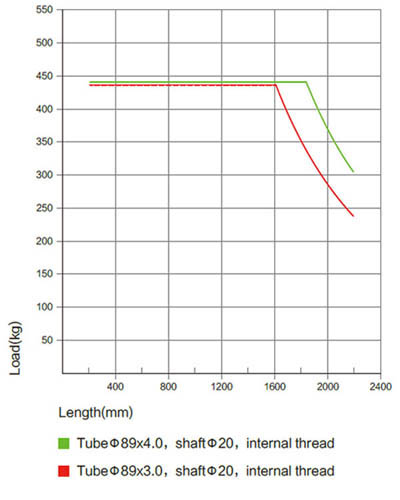Note: Above data shows the static load capacity of the roller for a uniformly distributed load.
You should also consider the chain tension, motor power drive factors, calculation based on the smallest value.

2411 / 2421 SERIES LOAD CAPACITY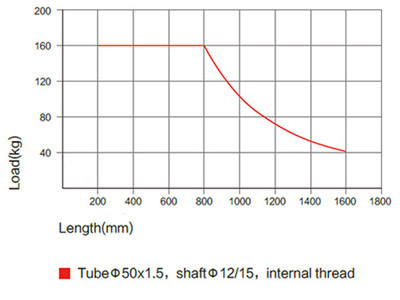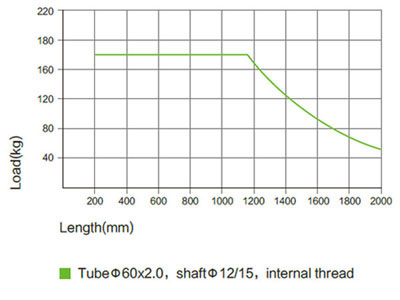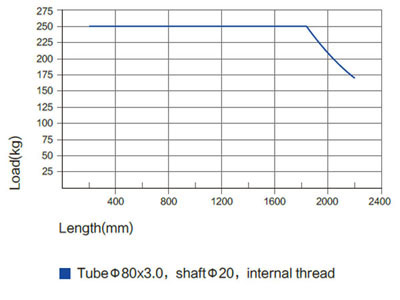Note: Above data shows the static load capacity of the roller for a uniformly distributed load.
You should also consider the chain tension, motor power drive factors, calculation based on the smallest value.